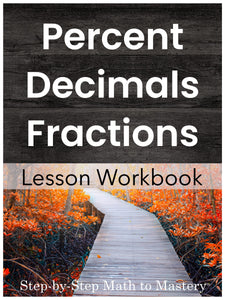# Converting Fractions, Decimals & Percents | Percent Word Problems

• \$10.00
Unit price per
Shipping calculated at checkout.

## Converting Fractions, Decimals and Percents with Simple Percentage Word Problems

### 13 Step-by-Step Math Lessons | Special Education Math Intervention | Fifth Grade Math Level

This Converting Between Fractions, Decimals, and Percent lesson workbook includes an example IEP goal, 13 step-by-step math lessons, reviews and assessments. This evidence-based math intervention is tied to fifth and sixth grade standards and is great for special education math goals and tier 2 small group math interventions (RTI).

This workbook is an introduction to percent.  Lessons teach how to convert between percent, fractions, and decimals.  Skills are introduced incrementally.  Scripted model problems make it easy to teach clear, sequential lessons.  By the end of these lessons, students will be able to solve simple percentage word problems

These sequential lessons are easy to teach, with enough material for 3 weeks of instruction!

★ Student Booklet Cover

★ 2 Lessons on Converting Fractions to Decimals

★ 1 Lesson on Converting a Percent to a Decimal

★ 3 Lessons on Finding a Percent of a Number & Checking to Make Sure it Makes Sense

★ 2 Lessons on Converting a Decimal to a Percent

★ 2 Lessons on Converting a Fraction to a Percent

★ 3 Lessons on Solving Word Problems

★ 2 Reviews & 2 Tests

108 Pages

3 Weeks of Instruction

## Students Will Be Able To:

• Convert a fraction to a decimal
• Round off repeating decimals
• Convert a percent to a decimal
• Find a percent “of” a number
• Solve word problems by finding the percent “of” a number
• Convert a decimal to a percent
• Convert a fraction to a percent (Round decimals to nearest hundredth)
• Solve word problems by converting a fraction to a percent

• Add, subtract, multiply, and divide decimals to hundredths, using concrete models or drawings and strategies based on place value, properties of operations, and/or the relationship between addition and subtraction; relate the strategy to a written method and explain the reasoning used. (CCSS 5.NBT.B.7)

• Fluently add, subtract, multiply, and divide multi-digit decimals using the standard algorithm for each operation. (CCSS 6.NS.B.3)

• Find a percent of a quantity as a rate per 100 (e.g., 30% of a quantity means 30/100 times the quantity); solve problems involving finding the whole, given a part and the percent. (CCSS 6.RP.A.3.c)

### Not sure where to start?

Watch a video overview of Step-by-Step Math to Mastery or read the transcript here.

### Step-by-Step Math to Mastery™ Resources:

Make math easier to understand.

• Help students over math hurdles with clear, sequential, scaffolded lessons.
• Prevent overwhelm. Build student confidence.

Make math easier to teach.

• Save hours of planning and piecing together materials.
• Paraeducators can deliver quality instruction independently. Open & teach.

### Reasons You'll Love Step-by-Step Math to Mastery™ Resources

• Boosts student confidence and progress
• Time-saving: print and teach
• Easy prep: only black ink is needed
• Easy to teach, paraeducator-friendly
• Example IEP goals and shorter term objectives
• Consistent & predictable format
• Lots of practice repetitions
• Scaffolded with structured workspaces
• Fewer problems on a page, white space, minimal visual clutter
• Tied to standards
• Explicit/Direct instruction
• Systematic & sequential
• Mastery approach--teaching one topic at a time, one strategy at a time
• Lessons have "I Can" statements, model problems, guided practice, & independent practice
• Each workbook can be used individually as a stand-alone intervention for that skill or they can be used together, taking students from the basics of number sense and addition in first grade all the way to dividing fractions and decimals in fifth grade.
• Can also be used with older students (middle & high school) to help fill the gaps in their learning.